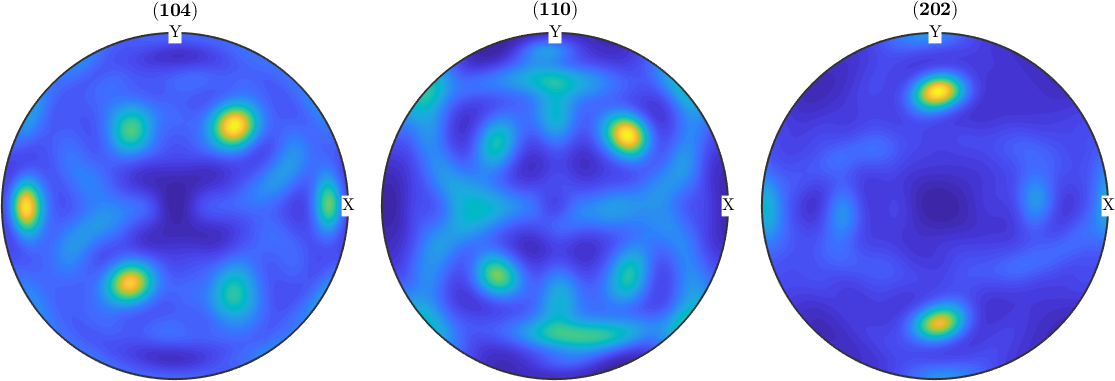Plotting of Pole Figures edit page

This section describes various possibilities to visualize pole figure data. Lets start by loading some XRD data

By default MTEX plots pole figures by drawing a circle at every measurement position of a pole figure and coloring it corresponding to the measured intensity.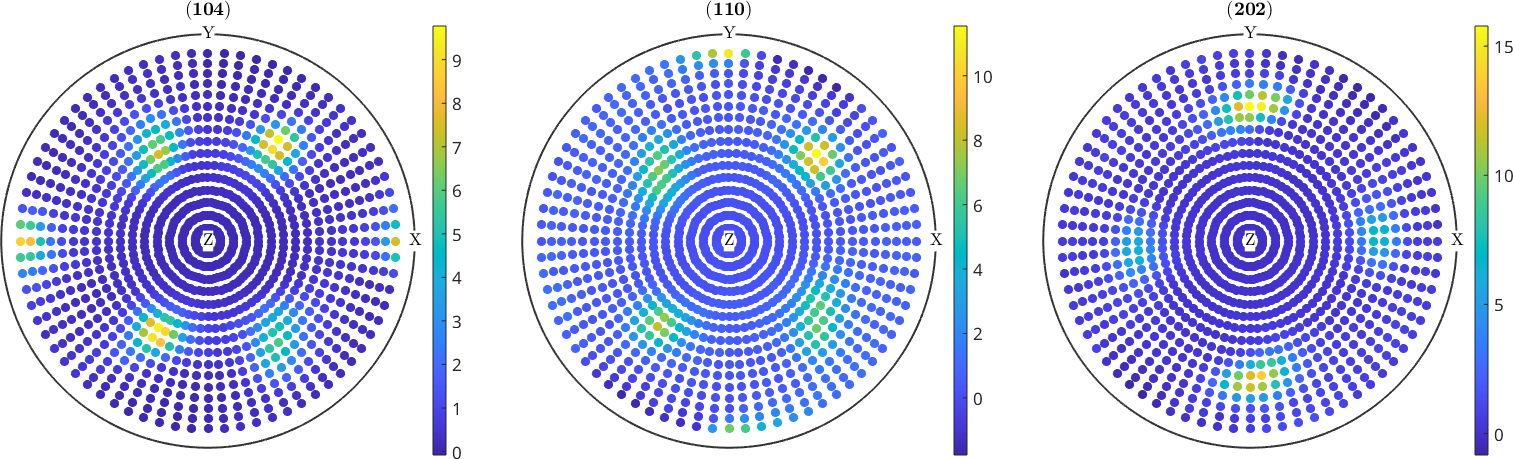MTEX tries to guess the right size of the circle in order to produce a pleasing result. However, you can adjust this size using the option MarkerSize.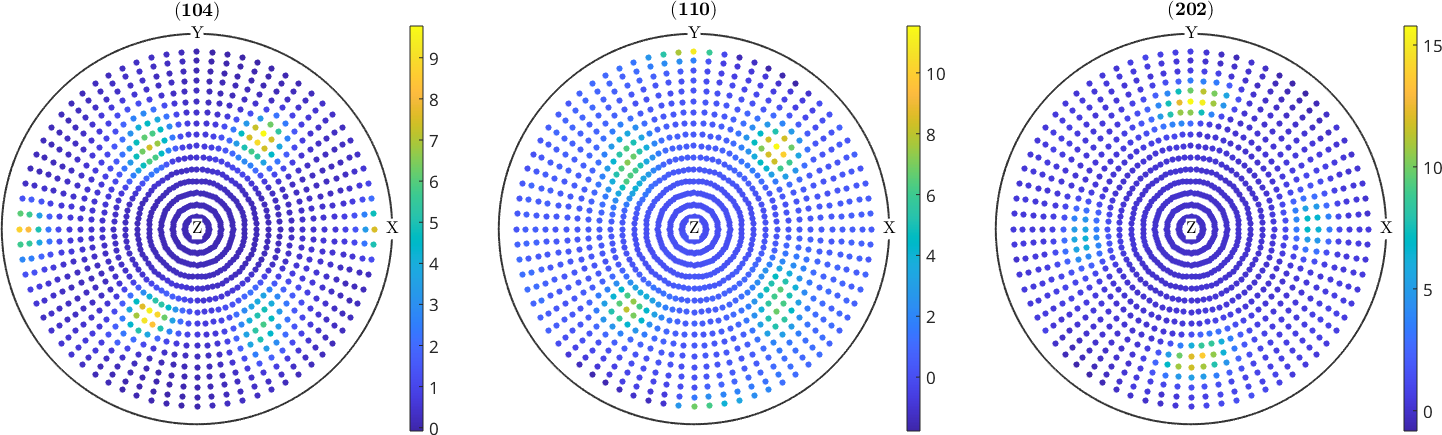## Contour Plots

Some people like to have their raw pole figures to be drawn as contour plots. This feature is not yet generally supported by MTEX. Note that measured pole figure may be given at a very irregular grid which would make it necessary to interpolate before drawing contours. In this case, however, it seems to be more reasonable to first compute an ODF and than to draw contour plots of the recalculated pole figures.

Nevertheless, MTEX offers basic contour plots in the case of regular measurement grids.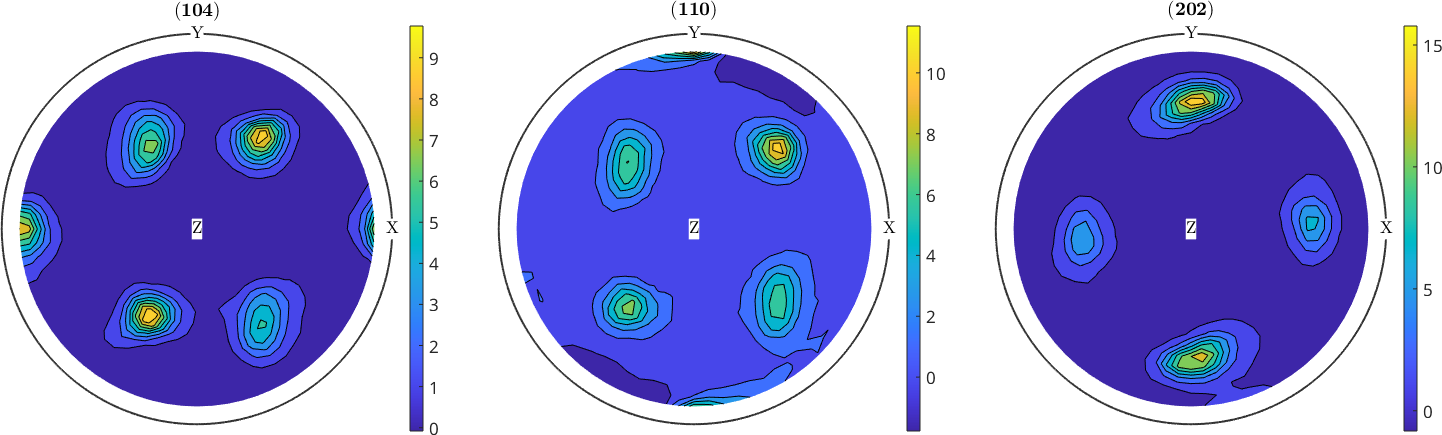When drawing a colorbar next to the pole figure plots it is necessary to have the same color coding in all plots. This can be done as following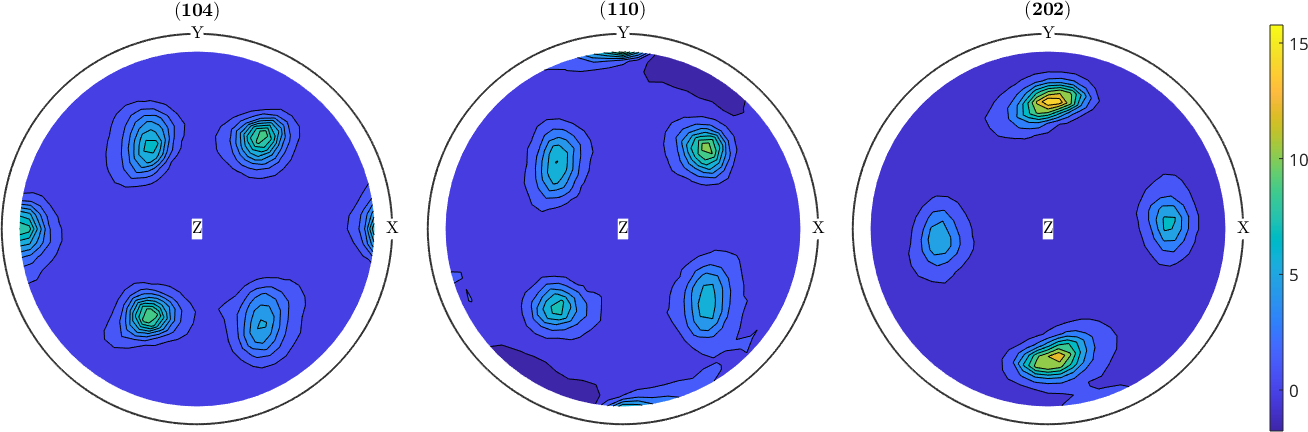## Plotting Recalculated Pole Figures

In order to draw recalculated one first needs to compute an ODF.

Now smooth pole figures can be plotted for arbitrary crystallographic directions.